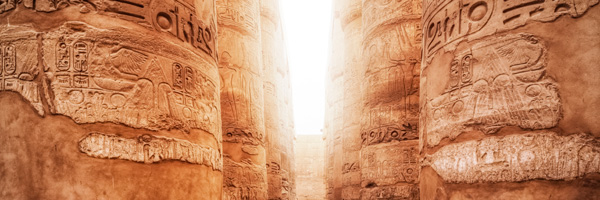# Egyptian FractionsA unit fraction is a fraction with a numerator of $1$, like $\frac{1}{3}$ or $\frac{1}{7}$or $\frac{1}{224}$.

An Egyptian Fraction is written as the sum of different unit fractions.

We can write $\frac{2}{3}$ and $\frac{3}{7}$ as Egyptian Fractions:

$\frac{2}{3}=\frac{1}{2} + \frac{1}{6}$

$\frac{3}{7} = \frac{1}{3} + \frac{1}{11} + \frac{1}{231}$.

I wonder whether all fractions can be written as Egyptian Fractions...

A good place to start investigating Egyptian Fractions is to explore whether all unit fractions can be written as the sum of two different unit fractions.

Here are two examples:

$\frac{1}{2} = \frac{1}{3} + \frac{1}{6}$.

$\frac{1}{6} = \frac{1}{7} + \frac{1}{42}$

Can you write other unit fractions as the sum of two unit fractions?

Can you write all unit fractions as the sum of two unit fractions?